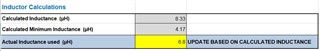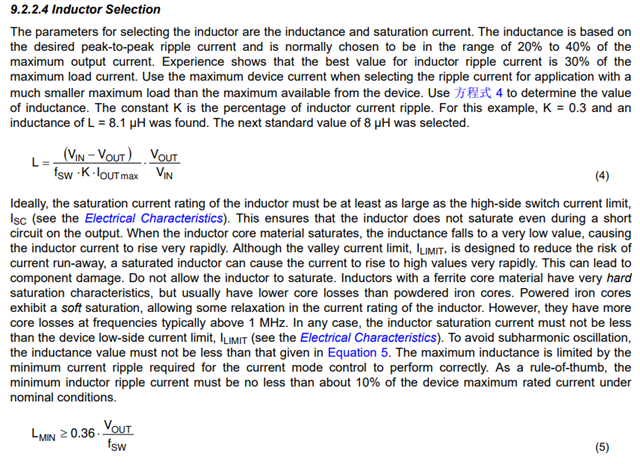If you have a related question, please click the "Ask a related question" button in the top right corner. The newly created question will be automatically linked to this question.

# LMR33610: What is the equation of this Inductor Calculation?

Part Number: LMR33610

Hi,,

I found the LMR336 calculator from TI, And i would like to know what formula is used in this Inductor calculation:Thank you for the help• Hi,

The formulas are shown below. You can find them in the datasheet of LMR33610. For the calculated inductance(uH), you can get 8.33uH by taking K in (4) to be 0.3.Thanks a lot.

Best Regards,

Aurora

• Hi Aurora,

Thank you so much, have a nice day